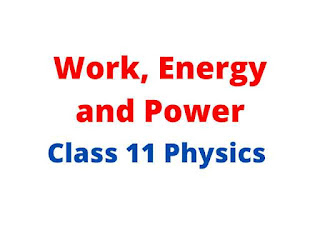# Work, Energy and Power Class 11 Notes pdf Nepal

0

In this blog I'm covering work, energy and power class 11 notes with pdf download. These notes contains complete questions and answers, formulas, and is made for self study purpose based on New syllabus of physics class 11.

This note contain:

• State and prove conservation of mechanical energy.
• Derive an expression to calculate work done by a variable force.
• Prove that the colliding objects exchange their velocities in one dimensional elastic collision.
• Definition of many important topics
• Complete numerical of work, energy and power
• Old is Gold solved questions
• and many more...work, energy and power class 11 physics

Work, energy and power is one of the important chapter of class 11 physics which contain more than 3 most important and most repeated questions for neb exam.

### What is Work?

A work is said to be done if the force applied on a body displaces it in the direction of force.

#### What is Energy?

It is defined as the capacity of doing work. There are two types of mechanical energy:
• Potential Energy : It is the energy possessed by a body due to its position from the ground.
• Kinetic Energy: It is defined as the energy possessed by a body when it is in motion.

#### What is Work - Energy theorem?

It states that work done on a body is equal to increase in kinetic energy of the body.

#### What is Principle of conservation of energy?

It states that energy can neither be created nor be destroyed but it simply changes its form from one to another.

#### What is Collision?

It is the mutual interaction between the two particles due to which their kinetic energy or momentum or path change. There are two types of collision:
Elastic collision: The collision in which total linear momentum and total kinetic energy of the system are conserved is called elastic collision. Example: collision between gas molecule.

#### Properties of elastic collision:

• Total linear momentum is conserved.
• Total kinetic energy is conserved.

1. scroll to the last of the article
3. Click to download button of article (this will redirect to drive (pdf))

## Download the complete pdf of numerical of Physics Class 11 from Here:

The above link is the download link of work, energy and power class 11 notes pdf. Click above and you will get redirected to the drive and then download the note by simply one click.

That's all what I can do for you guys. If this note series is helpful for you then please comment down #notes. This will motivate me a lot.

Also I gave complete Notes of Kinematics + Law of motion + Scalars and Vectors previously. Download all the notes from below: